Next: Syntax Up: Functional programming Previous: Outermost reduction and infinite   Contents

# The (untyped) lambda calculus

The lambda calculus was invented by Alonzo Church around 1930 as an attempt to formalize a notation for computable functions. It is important to have some basic familiarity with the lambda calculus because it forms the foundation for the definition of functional programming languages such as Haskell.

In the conventional set theoretic formulation of mathematics, a function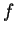can be represented by its graph--that is, a binary relation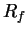between the sets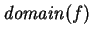and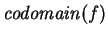consisting of all pairs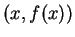. Not all binary relations represent functions--functions are single-valued, so if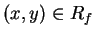andthen we must have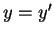. In mathematics, functions are almost always total, so we also have an additional requirement that for each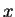in, there exists a pair. Representing a function in terms of its graph is called an extensional definition--two functions are equal if and only if their graphs are equal. This is analogous to the definition of equality in set theory--two sets are equal if and only if they have the same elements.

From a computational viewpoint, it is not sensible to represent functions merely in terms of graphs. Clearly, all sorting algorithms define functions that have the same graph. However, from a computational viewpoint, we would like to distinguish efficient sorting algorithms from inefficient ones.

What we need is an intensional representation of functions--a representation that captures not just the output value for each input, but also how this value is arrived at.

With this background, we plunge into the (pure untyped) lambda calculus.

SubsectionsNext: Syntax Up: Functional programming Previous: Outermost reduction and infinite   Contents